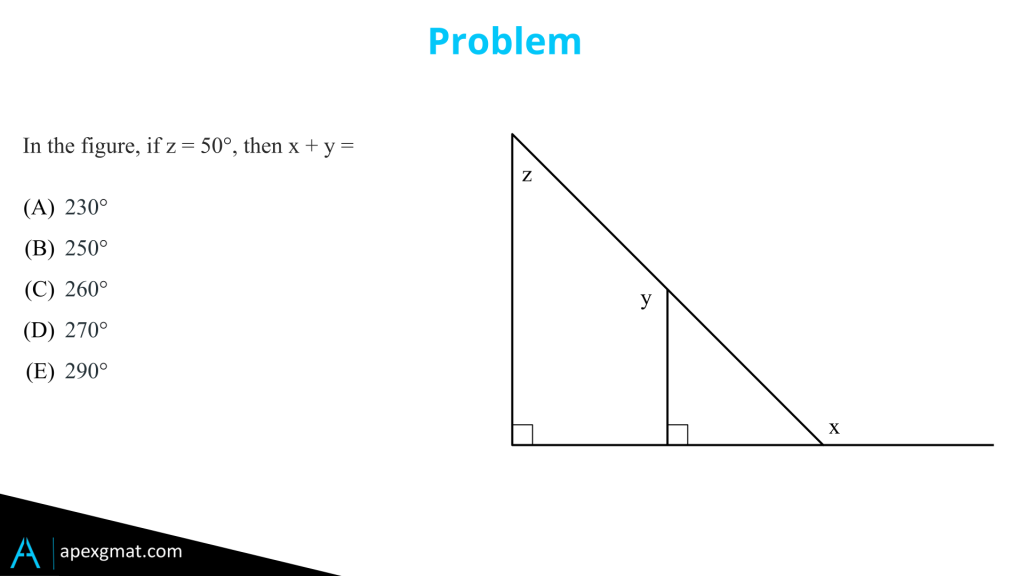## GMAT Geometry Problem

Hey guys, top level geometry problems are characterized typically by stringing a whole bunch of different rules together and understanding how one thing relates to the next thing, to the next thing. Until you get from the piece of information you started with to the conclusion. We’re going to start out by taking a look at this problem using the z equals 50° and seeing how that information goes down the line.But afterwards we’re going to see a super simple logical pathway utilizing a graphic scenario that makes the z equals 50° irrelevant. To begin with we’re being asked for the sum of x and y and this will come into play on the logical side. We need the sum not the individual amounts but let’s begin with the y. We have a quadrilateral and it has parallel sides which means the two angles z and y must equal 180°. That’s one of our geometric rules. If z is 50° that means y is 130° and we’re halfway there.

Next we need to figure out how x relates and there are several pathways to this. One way we can do it is drop. By visualizing or dropping a third parallel line down, intersecting x, so on the one hand we’ll have 90 degrees. We’ll have that right angle and on the other we’ll have that piece. Notice that the parallel line we dropped and the parallel line next to z are both being intersected by the diagonal line going through which means that that part of x equals z. So we have 50° plus 90° is 140°. 130° from the y, 140° from the x, gives us 270°.

Another way we can do this is by taking a look at the right triangle that’s already built in z is 50° so y is 1 30°. now the top angle in the triangle must then be 180° minus the 130° that is 50°. it must match the z again we have the parallel lines with the diagonal coming through then the other angle the one opposite x is the 180° degrees that are in the triangle minus the 90° from the right triangle brings us to 90° minus the 50° from the angle we just figured out means that it’s 40° which means angle x is 180° flat line supplementary angles minus the 40° gives us 140° plus the 130° we have from y again we get to 270°.

#### Graphic Solution Path

Now here’s where it gets really fun and really interesting. We can run a graphic scenario here by noticing that as long as we keep all the lines oriented in the same way we can actually shift the angle x up. We can take the line that extends from this big triangle and just shift it right up the line until it matches with the y. What’s going to happen there, is we’re going to see that we have 270° degrees in that combination of x and y and that it leaves a right triangle of 90°, that we can take away from 360° again to reach the 270°.

Here the 50° is irrelevant and watch these two graphic scenarios to understand why no matter how steep or how flat this picture becomes we can always move that x right up and get to the 270°. That is the x and y change in conjunction with one another as z changes. You can’t change one without the other while maintaining all these parallel lines and right angles. Seeing this is challenging to say the least, it requires a very deep understanding of the rules and this is one of those circumstances that really points to weaknesses in understanding most of what we learn in math class in middle school, in high school. Even when we’re prepping only scratches the surface of some of the more subtle things that we’re either allowed to do or the subtle characteristics of rules and how they work with one another and so a true understanding yields this very rapid graphic solution path.

#### Logical Solution Path

The logical solution path where immediately we say x and y has to be 270° no matter what z is and as you progress into the 80th, 90th percentile into the 700 level on the quant side this is what you want to look for during your self prep. You want to notice when there’s a clever solution path that you’ll overlook because of the rules. Understand why it works and then backtrack to understand how that new mechanism that you discovered fits into the framework of the rules that we all know and love. Maybe? I don’t know if we love them! But they’re there, we know them, we’re familiar with them, we want to become intimate. So get intimate with your geometry guys put on some al green light some candles and I’ll see you next time.

If you enjoyed this problem, try other geometry problems here: GMAT Geometry.